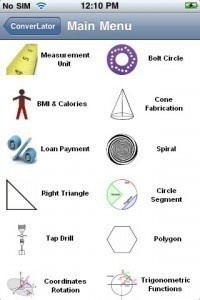# Measurements Converter

This is a measurements converter guide for changing commonly used units.This list also includes common cooking measurement conventions.

### US and Metric System: Length

1 centimeter is equal to 0.39 inches, while 1 meter is the same as 1.09 yards. 1 kilometer is equal to 0.62 miles. An inch is the same as 2.54 centimeters. A foot is equal to 30.48 centimeters and a yard is the same as 0.91 meters. A mile is equal to 1.61 kilometers.

### US and Metric System: Weight

A gram is the same as 0.035 ounces and a kilogram is the same as 2.21 pounds. The metric ton is about 1000 kg or 0.98 UK tons. An ounce is the same as 28.35 grams, while the pound’s metric equivalent is 0.45 kilograms. This measurements converter can also point out that the US ton is equal to 2000 pounds and 0.91 metric tons.

### US and Metric System: Area

1 acre is equal to 0.40 hectare while 1 hectare is equivalent to 2.47 acres. A square inch is the same as 6.45 square centimeters. A square centimeter is the same as 0.16 square inches. A square mile is the same as 2.60 square kilometers. A cubic feet is equal to 0.028 cubic meters and the cubic yard is 0.76 cubic meters in the metric system.

### Measurements Used in Cooking: Volume

A measurements converter for recipes show that 1 milliliter = 1/5 teaspoon, while 1 milliliter = 0.03 fluid ounce. A teaspoon is equal to 5 milliliters while a tablespoon is the same as 15 milliliters. 1 fluid ounce is equal to 30 milliliters.

A fluid cup is equal to 236.6 milliliters while a quart is the same as 946.4 milliliters. 1 liter is equal to 1000 milliliters or 34 fluid ounces. A liter is equivalent to 4.2 cups and also 2.1 fluid pints. The liter is also equal to 0.26 gallon and 1.06 fluid quarts. 3.8 liters is equal to one gallon.

### Recipes Measurements Converter: Weights

1 ounce is equal to 28.35 grams and 1 pound is the same as 453.59 grams. 1 gram is the same as 0.035 ounce. 100 grams is equal to 3.5 ounces and 1000 grams is 2.2 pounds. A kilogram is 35 ounces or 2.2 pounds.

### Temperature Conversion

The formula for converting C to F and F to C are C = (F – 32) X 5/9 and F = (C X 9/5) + 32. Some common Celsius to Fahrenheit measurements are the following; 32F = 0C, 40F = 4.4C, 100F = 37.7C, 200F = 93.3C, 225F = 107.2C, 250F = 121.1C and 275F = 135C.

### Unique Weights and Measures

1 bit = 2 pinches while 1 smidgen = 4 bits. 1 dollop = 2 smidgens and 1 gaggle = 3 dollops. 1 gaggle = 2 glugs and 1 blanket = 2 glugs.

Whether you are traveling or doing research on the Internet, a measurements converter can be a valuable resource. It will eliminate a lot of problems when it comes to figuring various lengths and weights.